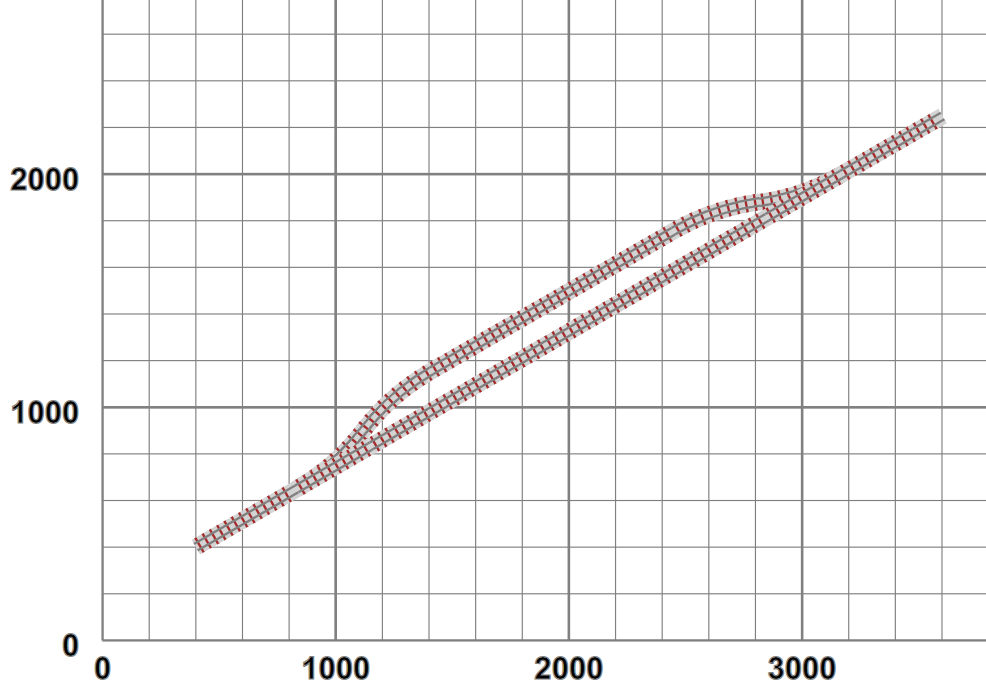## Drawing pictures

Thus far we have drawn schematic representations of the track as SVG line-based components. With a little work SVG is entirely capable of generating much more detailed views, with a lot of possibility of caching intermediate and reused sections. For example:

Figure 10. More detailed trackIn this case the track is generated from a sequence of rail-and-sleeper subsection definitions, displayed via SVG's `use` directive:

```<g xmlns="http://www.w3.org/2000/svg" class="way"
transform="translate(3769.549241302635,2599) rotate(30)">
<use href="#track10" x="0" y="0"/>
<rect class="ballast" x="360" y="-36" width="140" height="72"/>
<use href="#sleeper" x="378" y="0"/>
<use href="#sleeper" x="414" y="0"/>
<use href="#sleeper" x="450" y="0"/>
<line class="rail  SM32" x1="360" y1="-16" x2="500" y2="-16"/>
<line class="rail  SM32" x1="360" y1="16" x2="500" y2="16"/>
</g>```

In this case we have a pre-built 10-sleeper section of straight track (`#track10`), followed by ballast, three sleepers and two rails to display the remainder of the required length. All these are sized to the actual dimensions of the track being used. This is translated and rotated into the required start position.